﻿ Effect of Tine Depth and Width on Soil Failure Angle, Cutting Coefficients and Power in Three-Dimensional Case: Computer Modeling

### Effect of Tine Depth and Width on Soil Failure Angle, Cutting Coefficients and Power in Three-Dimens...

Moayad B. Zaied, Ahmed M. El Naim, Omer A. Abdalla, Abuobieda M. Sulieman

World Journal of Agricultural Research

## Effect of Tine Depth and Width on Soil Failure Angle, Cutting Coefficients and Power in Three-Dimensional Case: Computer Modeling

Moayad B. Zaied1, Ahmed M. El Naim2,, Omer A. Abdalla3, Abuobieda M. Sulieman4

1Department of Agricultural Engineering, Faculty of Natural Resources and Environmental Studies, University of Kordofan, Elobied, Sudan

2Department of Crop Sciences, Faculty of Natural Resources and Environmental Studies, University of Kordofan, Elobied, Sudan

3Department of Agricultural Engineering, Faculty of Agriculture, University of Khartoum, Khartoum, Sudan

4Department of Mechanical Engineering, Faculty of Engineering and Technical Studies, University of Kordofan, Elobied, Sudan

### Abstract

The dynamic response of soil to farm implements is a main factor in determining their performance. The interaction between tillage tools and soil is of a primary interest to the design and use of these tools for soil manipulation. A computer simulation is conducted by developing a program using C ++ programming language to study effect of tool depth and width on angle of soil failure plane, soil cutting coefficients, soil resistance force and Power requirements in three-dimensional soil cutting. The results demonstrated that at 0.2 m tine depth the highest values of angle of soil failure plane, frictional coefficient, overburden coefficient, soil cohesion coefficient and soil adhesion coefficient were 52.6°, 19.8, 49.54, 16.47 and 1.38 respectively and they were recorded by 0.04 m tine width while the lowest values in same sequence were 51.6°, 10.64, 22.05, 7.26 and 1.30 and they were recorded by 0.1 m width. The lowest values of soil resistance force and power were 1.77 kN and 1.03 kW and they were shown by 0.04 m width while the highest values were 2.07 kN and 2.26 kW and they were demonstrated by 0.1 m width of tine. At 0.3 m tine depth the highest values of angle of soil failure plane, frictional coefficient, overburden coefficient, soil cohesion coefficient and soil adhesion coefficient were 52.7°, 27.42, 72.54, 24.11 and 1.39 respectively and they were recorded by 0.04 m tine width while the lowest values in same sequence were 52.3°, 13.70, 31.23, 10.35 and 1.35 and they were recorded by 0.1 m width. The lowest values of soil resistance force and power were 4.27 kN and 4.66 kW and they were shown by 0.04 m width while the highest values were 4.86 kN and 5.29 kW and they were demonstrated by 0.1 m width of tine. At 0.4 m tine depth the highest values of angle of soil failure plane, frictional coefficient, overburden coefficient, soil cohesion coefficient and soil adhesion coefficient were 52.8°, 35.04, 95.27, 31.73 and 1.39 respectively and they were recorded by 0.04 m tine width while the lowest values in same sequence were 52.5°, 16.75, 40.39, 13.40 and 1.37 and they were recorded by 0.1 m width. The lowest values of soil resistance force and power were 8.19 kN and 8.93 kW and they were shown by 0.04 m width while the highest values were 9.13 kN and 9.95 kW and they were demonstrated by 0.1 m width of tine. It was concluded that Angle of soil failure plane and soil cutting coefficients decreased as tine working depth and width increased. Soil resistance force and power increased as angle of soil failure plane and soil cutting coefficients decreased as tine working depth and width increased.

• Moayad B. Zaied, Ahmed M. El Naim, Omer A. Abdalla, Abuobieda M. Sulieman. Effect of Tine Depth and Width on Soil Failure Angle, Cutting Coefficients and Power in Three-Dimensional Case: Computer Modeling. World Journal of Agricultural Research. Vol. 4, No. 1, 2016, pp 24-30. http://pubs.sciepub.com/wjar/4/1/4
• Zaied, Moayad B., et al. "Effect of Tine Depth and Width on Soil Failure Angle, Cutting Coefficients and Power in Three-Dimensional Case: Computer Modeling." World Journal of Agricultural Research 4.1 (2016): 24-30.
• Zaied, M. B. , Naim, A. M. E. , Abdalla, O. A. , & Sulieman, A. M. (2016). Effect of Tine Depth and Width on Soil Failure Angle, Cutting Coefficients and Power in Three-Dimensional Case: Computer Modeling. World Journal of Agricultural Research, 4(1), 24-30.
• Zaied, Moayad B., Ahmed M. El Naim, Omer A. Abdalla, and Abuobieda M. Sulieman. "Effect of Tine Depth and Width on Soil Failure Angle, Cutting Coefficients and Power in Three-Dimensional Case: Computer Modeling." World Journal of Agricultural Research 4, no. 1 (2016): 24-30.

 Import into BibTeX Import into EndNote Import into RefMan Import into RefWorks

12345
Prev Next

### 1. Introduction

Limit equilibrium is one of the most important approaches used to analyze soil–tool systems. Two most important factors in the approach are the shape of soil failure surface, and equilibrium equations, which are two or three dimensional cases. Several mathematical models have been developed for predicting the performance characteristics of tillage tools in soils. These include two-dimensional models for wide tools and three dimensional models for narrow tillage tools. Payne's model  was the first three-dimensional soil failure model By observing the top surface soil heave during tillage, a failure zone was proposed The failure zone included a triangular centre wedge, a centre crescent, and two side blocks (called wings of the crescent).

Extensive tests were conducted by Payne and Tanner  to study the effects of the failure zone size and the rake angle on the draft force. However, no equations were developed to evaluate the draft force for narrow tillage tools. The analysis of three-dimensional failure assumes that the failure configuration is composed of forward and sideways failure regimes .

Tillage tool performance under two different soil conditions has been predicted using the four models. Simulated results are compared with the experimental results . The Godwin-Spoor  developed a model to predict forces on narrow tillage tines with a wide range of depth to- width ratios.

The three-dimensional model developed by McKyes and Ali  is similar to the Godwin-Spoor model, one major difference is that the McKyes-Ali model does not require prior knowledge of the rupture distance (r) for computing the forces on the tool. Also Perumpral et al.  developed a model similar to models developed by McKyes and Ali  and Godwin and Spoor . However, the side wedges flanking the center wedge were replaced by two sets of forces acting on the sides of the center wedge. Swick and Perumpral  proposed a dynamic soil cutting model which included the effect of travel speed. The failure zone of the model, similar to the McKyes-Ali model, consisted of a centre wedge and two side crescents with a straight rupture plane at the bottom.

Zeng and Yao  developed another dynamic soil cutting model which included the acceleration and damping effect on the basis of their studies on the relation between soil shear strength and shear strain rate and the relation between soil-metal friction and sliding speed. The failure zone of the model was similar to that of the McKyes-Ali. model. One major difference between these two models is that the Zeng-Yao model requires prior knowledge of failure shear strain for determining the position of the shear failure boundary.

Chung, and. Sudduth  introduced mathematical models to estimate the force required to penetrate (cut and displace) soil with a prismatic cutter traveling horizontally and with a cone penetrometer traveling vertically were developed based on the passive earth pressure theory and the concept of a variable failure boundary. Both models were expressed as additive forms of density, cohesion, and adhesion components of the soil, with each effect multiplied by a corresponding dimensionless number. Zaied et al  developed an explicit mathematical model for angle of soil failure plane in case of three dimensional cutting. Tagar et al  reported that FEM provided acceptable simulation of soil failure patterns and it was also shown while the internal friction angle was not affected by any failure pattern in either soil bin or field conditions. Mari et al  concluded that deformations of soil force have direct relationship with speed and depth with dominant effect of depth and inverse relation with moisture content and draught forces also exhibit similar trend with speed and depth.

The objective of present study is conduct a computer modeling to predict the effect of depth and width of narrow tine on the angle of soil failure plane, soil cutting coefficients and power requirement in case of three dimensional soil cutting analysis using equation 1 of soil failure developed by Zaied et al .

### 2. Materials and Methods

The computer modeling is conducted by developing a program using C + + programming language. Mathematical models used are as follows: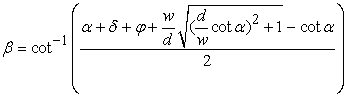(1)

Where,= tool rake angle (deg.),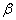= angle of soil failure plane (deg.),= angle of external soil friction (deg.),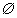= angle of internal soil friction (deg.),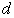= tool operating depth (m),= tool operating width (m).(2)(3)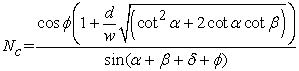(4)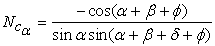(5)

Where,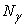= soil friction cutting coefficient (dimensionless),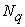= soil overburden cutting coefficient (dimensionless),= soil cohesion cutting coefficient (dimensionless),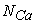= soil adhesion cutting coefficient (dimensionless).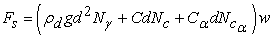(6)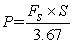(7)

Where

FS = soil resistance force (kN), C = soil cohesion (kPa), Ca = soil adhesion (kPa), g = acceleration due to gravity (m / s2),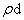= soil bulk density (g / cm3), P = power (kW), S = operating speed (km / h).

Inputs of soil and tine variables are shown in Table 1

#### Table 1. Soil and tine inputs

The inputs were used in a program developed in C + + language, the program was then built and executed to predict angle of soil failure plane, soil frictional cutting coefficient, soil overburden cutting coefficient, soil cohesion cutting coefficient, soil adhesion cutting coefficient, soil resistance force and power. These variables were predicted at different values of working depth and width of tines.

### 3. Results and Discussion

The flowchart of the program is shown in Figure 1 while effect of tine operating depth and width on angle of soil failure plane, soil frictional coefficient, soil overburden coefficient, soil cohesion coefficient, soil adhesion coefficient, soil resistance force and power was shown in Table 2, Table 3 and Table 4.Download asVeiw figureFigures index
Figure 1. The flowchart of the program

#### Table 2. Angle of soil failure plane, soil cutting coefficients, and power requirements at 0.20 m depthDownload asVeiw figureFigures index
Figure 2. Effect of tine width on angle of soil failure plane at 0.2 m depthDownload asVeiw figureFigures index
Figure 3. Effect of tine width on soil cutting coefficients at 0.2 m depth

At 0.2 m tine depth (Table 2), the value of angle of soil failure plane decreased from 52.6° as a maximum value at 0.04 m width to a minimum value of 51.6° at 0.1 m width (Figure 2). Soil frictional coefficient was decreased from 19.8 to 10.64 at 0.04 m and 0.1 m respectively, in case of overburden coefficient, the highest value was 49.54 and it was recorded at 0.04 m width while the lowest value was 22.05 and it was recorded at 0.1 m width, the highest value of soil cohesion coefficient was 16.47 while the lowest value was 7.26 and they were demonstrated by 0.04 m and 0.1 m width respectively, the maximum value of soil adhesion coefficient was 1.38 and it was recorded by 0.04 m width while the minimum value was 1.30 and it was shown by 0.1 m depth (Figure 3). Soil resistance force and power found to be increased as tine operating width increased, the lowest and highest values of soil resistance force were 1.77 kN (Figure 4) and 2.07 kN (Figure 5) and they were recorded by 0.04 m and 0.1 m width respectively. The lowest value of power was 1.03 kW and it was recorded by 0.04 m depth while the highest value was 2.26 kW and it was demonstrated by 0.1 m depth of tine.Download asVeiw figureFigures index
Figure 4. Effect of tine width on soil resistance force at 0.2 m depthDownload asVeiw figureFigures index
Figure 5. Effect of tine width on power at 0.2 m depth

#### Table 3. Angle of soil failure plane, soil cutting coefficients, and power requirements at 0.30 m depthDownload asVeiw figureFigures index
Figure 6. Effect of tine width on angle of soil failure plane at 0.3 m depthDownload asVeiw figureFigures index
Figure 7. Effect of tine width on soil cutting coefficients at 0.3 m depth

At 0.3 m tine depth (Table 3), the value of angle of soil failure plane (Figure 6) decreased from 52.7° as a maximum value at 0.04 m width to a minimum value of 52.3° at 0.1 m width. Soil frictional coefficient was decreased from 27.42 to 13.70 at 0.04 m and 0.1 m respectively, in case of overburden coefficient, the highest value was 72.40 and it was recorded at 0.04 m width while the lowest value was 31.23 and it was recorded at 0.1 m width, the highest value of soil cohesion coefficient was 24.11 while the lowest value was 10.35 and they were demonstrated by 0.04 m and 0.1 m width respectively, the maximum value of soil adhesion coefficient was 1.39 and it was recorded by 0.04 m width while the minimum value was 1.35 and it was shown by 0.1 m depth (Figure 7). Soil resistance force and power found to be increased as tine operating width increased, the lowest and highest values of soil resistance force were 4.26 kN (Figure 8) and 4.86 kN (Figure 9) and they were recorded by 0.04 m and 0.1 m width respectively. The lowest value of power was 4.66 kW and it was recorded by 0.04 m depth while the highest value was 5.29 kW and it was demonstrated by 0.1 m depth of tine.Download asVeiw figureFigures index
Figure 8. Effect of tine width on soil resistance force at 0.3 m depthDownload asVeiw figureFigures index
Figure 9. Effect of tine width on power at 0.3 m depth

#### Table 4. Angle of soil failure plane, soil cutting coefficients, and power requirements at 0.40 m depth

At 0.4 m tine depth (Table 4), angle of soil failure plane (Figure 10) decreased from 52.7° as a maximum value at 0.04 m width to a minimum value of 52.5° at 0.1 m width. Soil frictional coefficient was decreased from 35.04 to 16.75 at 0.04 m and 0.1 m respectively, in case of overburden coefficient, the highest value was 95.27 and it was recorded at 0.04 m width while the lowest value was 40.39 and it was recorded at 0.1 m width, the highest value of soil cohesion coefficient was 31.73 while the lowest value was 13.42 and they were demonstrated by 0.04 m and 0.1 m width respectively, the maximum value of soil adhesion coefficient was 1.39 and it was recorded by 0.04 m width while the minimum value was 1.37 and it was shown by 0.1 m depth (Figure 11).Download asVeiw figureFigures index
Figure 10. Effect of tine width on angle of soil failure plane at 0.4 m depthDownload asVeiw figureFigures index
Figure 11. Effect of tine width on soil cutting coefficients at 0.4 m depthDownload asVeiw figureFigures index
Figure 12. Effect of tine width on soil resistance force at 0.4 m depth

Soil resistance force and power found to be increased as tine operating width increased, the lowest and highest values of soil resistance force were 8.19 kN (Figure 12) and 9.13 kN (Figure 13) and they were recorded by 0.04 m and 0.1 m width respectively. The lowest value of power was 8.93 kW and it was recorded by 0.04 m depth while the highest value was 9.95 kW and it was demonstrated by 0.1 m depth of tine.Download asVeiw figureFigures index
Figure 13. Effect of tine width on power at 0.4 m depth

The results showed that as tine operating width increased from 0.04 m to 0.1 m at 0.2 m, 0.3 m and 0.4 m depths, the angle of soil failure plane, soil frictional, overburden, cohesion and adhesion coefficients decreased. It was found that soil resistance force and power increased as tine working depth and width increased and angle of soil failure plane and soil cutting coefficients decreased.

### 4. Conclusion

A computer model was developed in C++ programming language to investigate the effect of width and depth of narrow tine on the angle of soil failure plane and power requirement in case of three dimensional soil cutting analysis.

Angle of soil failure plane and soil cutting coefficients decreased as tine working depth and width increased.

Soil resistance force and power increased as angle of soil failure plane and soil cutting coefficients decreased as tine working depth and width increased.

### References

  Payne, P.C. “The relationship between the mechanical properties of soil and the performance of simple cultivation implements£”. J. Agric. Eng. Res. l (l), 23-50, 1956.In article  Payne, P.C. and Tanner, D.W. “The relationship between rake angle and the performance of simple cultivation implements”. J. Agric. Eng. Res. 4(4),312-325, 1959.In article  Hettiaratchi, DR. P. and Reece, A.R. “Symmetrical three-dimensional soil failure”. J. Terramech. 4(3),45-67, 1967.In article  Grisso, R.D. and John V.P. “Review of Models for Predicting Performance of Narrow Tillage Tool”. American Society of Agricultural Engineers, 28(4), 1062-1067, 1985.In article  Godwin, R.J. and Spoor, G. “Soil failure with narrow tines”. J. Agric. Eng. Res. 22(4), 213-228. 1977.In article  McKyes, E. and Ali, O. S. “The cutting of soil by narrow blades”. J. Terramech. 14(2),43-58, 1977.In article  Perumpral, J.V., Grisso, R. D. and Desai, C. S. “A soil tool model based on limit equilibrium analysis”. TRANSACTIONS of the ASAE, 26(4), 991-995, 1983.In article  Swick, W.C. and Perumpral, J.V. “A model for predict ing soil-tool interaction”. Journal of Terramechanics, 25(l), 43-56, 1988.In article  Zeng, D. and Yao, Y. “A dynamic model for soil cutting by blade and tine”. Journal of Terramechanics, 29(3), 317-328, 1992.In article  Chung, S.O. and Sudduth, K.A. “Soil failure models for vertically operating and horizontally operating strength sensors”. American Society of Agricultural and Biological Engineers, 49(4): 851-863, 2006.In article  Zaied, M.B., Dahab, M.H., El Naim, A.M. “Development of a mathematical model for angle of soil failure plane in case of 3-dimensional cutting”. Current Research in Agricultural Sciences, 1(2), 42-52, 2014.In article  Tagar, A.A., J. Changying, J. Adamowski, J. Malard, C. S. Qi a, D. Qishuoand N.A. Abbasi. “Finite element simulation of soil failure patterns under soil bin and field testing conditions”. Soil & Tillage Research 145(2015), 157-170, 2015.In article  Mari, I. A., J. Changying1, N. Leghari, B. G. Ali, C. F. Ali, C. Arslan, A. Sattar, and F. Huimin. “Analyses of 3-dimensional draught and soil deformation forces caused by mouldboard plough in clay loam soil”. Global Advanced Research Journal of Agricultural Science. 4(6) pp. 259-269, 2015.In article